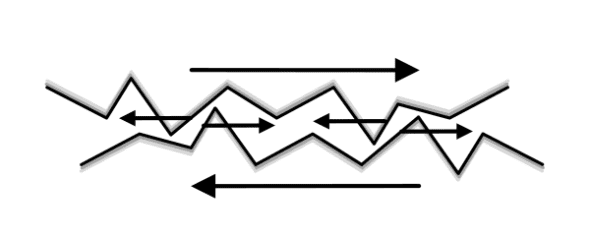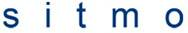# Basics of friction

ellomoto135
This is not necessarily a homework problem, as I am not in the course yet, but could someone please explain to me about the basics of friction

soothsayer

Sure, it's a pretty simple concept. To understand friction, you need to understand something called the "normal force" Imagine you are standing on a floor, obviously, gravity from the Earth is pulling down on you, but why aren't you accelerating? Because the floor provides a counter force to hold you in place. Similarly, imagine you put your hands on a wall and push on it, neither the wall nor you move anywhere because the wall provides enough counter force to hold you back. This kind of reactionary force that acts parallel to a surface is called the "normal force" and the strength of this force is one of the important factors in determining the strength of frictional forces. All real surfaces are not perfectly smooth, but instead have many irregularities on a visible or at least microscopic level. This means that any two surfaces sliding against each other will not slide perfectly smoothly, and will in fact apply a force on this other. On a microscopic level, this interaction might look something like this:This is the cause of the frictional force, it always acts in opposite direction as motion (as to provide a reactionary force as called for in Newton's third law, and the formula for finding the strength of this force is as follows:Where Fk is the frictional force acting on an object, Fn is the amount of normal force acting on the object, as described above, and
$$\mu$$k is called the "coefficient of friction", this number is different based on the surfaces that are interacting. As you well know, some surfaces provide more friction than other (ex: rubber has a higher coefficient of friction than oil).

This type of friction I described is called "kinetic friction" (which is why there is a small k in front of the F and mu in the above equation) and happens whenever two surfaces are rubbing against each other. Another kind of friction is called "static friction" this is friction that acts on an object resting on a surface with friction which keeps the object from moving. If you put you hand down on a table, desk, etc. You'll notice that it takes a certain amount of force to get it to start moving across that surface, this is because of static friction, which is actually larger than kinetic friction. This equation for static friction is the same as for kinetic friction, except, instead of using the coefficient of kinetic friction, you must use the coefficient of static friction which also depends on the surfaces creating the friction.

Those are the basics!